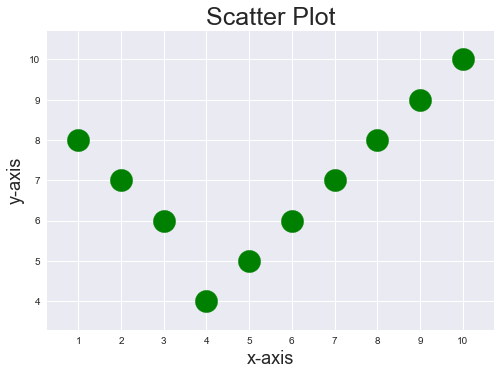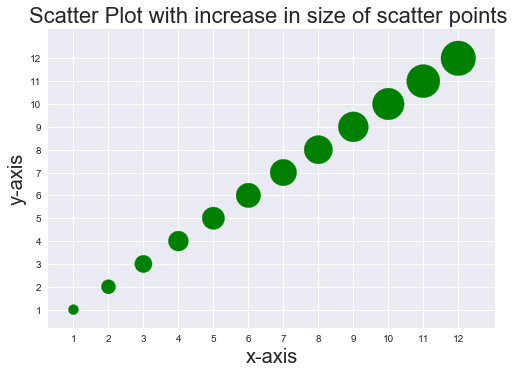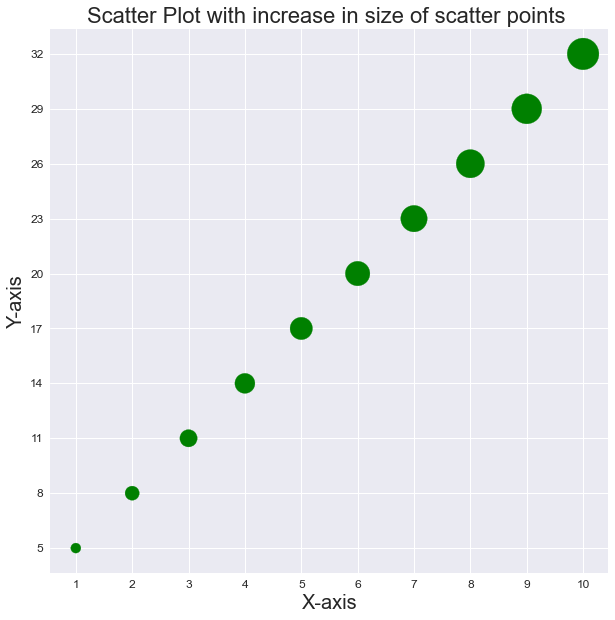Related Articles

# How to increase the size of scatter points in Matplotlib ?

• Difficulty Level : Medium
• Last Updated : 03 Jan, 2021

Prerequisites: Matplotlib

Scatter plots are the data points on the graph between x-axis and y-axis in matplotlib library. The points in the graph look scattered, hence the plot is named as ‘Scatter plot’. The points in the scatter plot are by default small if the optional parameters in the syntax are not used. The optional parameter ‘s’ is used to increase the size of scatter points in matplotlib. Discussed below are various ways in which s can be set.

Syntax :

matplotlib.pyplot.scatter(x_axis_data, y_axis_data, s=None, c=None, marker=None, cmap=None, vmin=None, vmax=None, alpha=None, linewidths=None, edgecolors=None)

Parameters:

• x_axis_data- An array containing x-axis data
• y_axis_data- An array containing y-axis data
• s- marker size (can be scalar or array of size equal to size of x or y)
• c- color of sequence of colors for markers
• marker– marker style
• cmap- cmap name
• linewidths- width of marker border
• edgecolor- marker border color
• alpha- blending value, between 0 (transparent) and 1 (opaque)

### Approach

• Import module
• Create data
• Set value for s
• Plot scatter plot
• Display plot

The parameter s can be set in multiple ways, it can be fixed value and it can also be a variable. When s is set to a variable values, data points on the scatter plot are of different sizes. Implementation is given below:

Example 1: Data points in scatter plot with an increased fixed size

## Python3

 `import` `matplotlib.pyplot as plt ` `import` `numpy as np ` ` `  `plt.style.use(``'seaborn'``) ` ` `  `x ``=` `[``1``, ``2``, ``3``, ``4``, ``5``, ``6``, ``7``, ``8``, ``9``, ``10``] ` `y ``=` `[``8``, ``7``, ``6``, ``4``, ``5``, ``6``, ``7``, ``8``, ``9``, ``10``] ` ` `  `plt.xticks(np.arange(``11``)) ` `plt.yticks(np.arange(``11``)) ` ` `  `plt.scatter(x, y, s``=``500``, c``=``'g'``) ` ` `  `plt.title(``"Scatter Plot"``, fontsize``=``25``) ` ` `  `plt.xlabel(``'x-axis'``, fontsize``=``18``) ` `plt.ylabel(``'y-axis'``, fontsize``=``18``) ` ` `  `plt.show() `

Output:Example 2: Data points in scatter plot with variable size

## Python3

 `import` `matplotlib.pyplot as plt ` `import` `numpy as np ` ` `  `plt.style.use(``'seaborn'``) ` ` `  `x ``=` `[``1``,``2``,``3``,``4``,``5``,``6``,``7``,``8``,``9``,``10``,``11``,``12``] ` `y ``=` `[``1``,``2``,``3``,``4``,``5``,``6``,``7``,``8``,``9``,``10``,``11``,``12``] ` `points_size ``=` `[``100``,``200``,``300``,``400``,``500``,``600``,``700``,``800``,``900``,``1000``,``1100``,``1200``] ` ` `  ` `  `plt.xticks(np.arange(``13``)) ` `plt.yticks(np.arange(``13``)) ` ` `  `plt.scatter(x,y,s``=``points_size,c``=``'g'``) ` ` `  `plt.title(``"Scatter Plot with increase in size of scatter points "``, fontsize``=``22``) ` ` `  `plt.xlabel(``'x-axis'``,fontsize``=``20``) ` `plt.ylabel(``'y-axis'``,fontsize``=``20``) ` ` `  `plt.show()`

Output:Example 3:

## Python3

 `import` `matplotlib.pyplot as plt ` ` `  `plt.style.use(``'seaborn'``) ` `plt.figure(figsize``=``(``10``, ``10``)) ` ` `  `x ``=` `[``1``, ``2``, ``3``, ``4``, ``5``, ``6``, ``7``, ``8``, ``9``, ``10``] ` `y ``=` `[``3``*``i``+``2` `for` `i ``in` `x] ` `size ``=` `[n``*``100` `for` `n ``in` `range``(``1``, ``len``(x)``+``1``)] ` `# print(size) ` ` `  `plt.scatter(x, y, s``=``size, c``=``'g'``) ` `plt.title(``"Scatter Plot with increase in size of scatter points "``, fontsize``=``22``) ` ` `  `plt.xlabel(``'X-axis'``, fontsize``=``20``) ` `plt.ylabel(``'Y-axis'``, fontsize``=``20``) ` ` `  `plt.xticks(x, fontsize``=``12``) ` `plt.yticks(y, fontsize``=``12``) ` ` `  `plt.show() `

Output:Attention geek! Strengthen your foundations with the Python Programming Foundation Course and learn the basics.

To begin with, your interview preparations Enhance your Data Structures concepts with the Python DS Course. And to begin with your Machine Learning Journey, join the Machine Learning – Basic Level Course

My Personal Notes arrow_drop_up
Recommended Articles
Page :